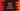# Python program to check if a year is a leap year or not## Python 3 program to check if a year is a leap year or not :

To check if a year is a leap year or not, we need to check if it is divisible by 4 or not. A year is a leap year if it is divisible by 4 and for century years if it also divisible by 400.

Following is the algorithm we are using in the example program :

### Steps to check if a year is a leap year or not :

1. Check if it is divisible by 4. If not then it is not a leap year.
2. If divisible by 4, check if it is divisible by 100. If not, it is not a century year, so it is a leap year.
3. If divisible by 100, check if it is divisible by 400 or not. If yes it is a leap year, else not. So, a leap year should be divisible by 4, 100 and 400. Else, it is not a leap year.

List of leap years from 1900 to 2020 are : 1904, 1908, 1912, 1916, 1920, 1924, 1928, 1932, 1936, 1940, 1944, 1948, 1952, 1956, 1960, 1964, 1968, 1972, 1976, 1980, 1984, 1988, 1992, 1996, 2000, 2004, 2008, 2012, 2016, 2020 .

Note that if a year is exactly divisible by 4, it is a leap year. But century years or years that are completely divisible by 100 are leap year only if they are exactly divisible by 400. For example, the years 1600 and 2000 are leap years but the years 1700, 1800 and 1900 are not leap year.

We will add all these conditions in our program below.

You can verify the above years with the following example :

### Program :

``````def printLeapYear():
print("Inpur Year is a Leap Year")

def printNotLeapYear():
print("Inpur Year is not a Leap Year")

input_year = int(input("Enter a Year : "))

if input_year % 4 == 0:
if input_year % 100 == 0 :
if input_year % 400 == 0 :
printLeapYear()
else :
printNotLeapYear()
else :
printLeapYear()
else :
printNotLeapYear()``````

### Sample Output :

``````Enter a Year : 2000
Inpur Year is a Leap Year

Enter a Year : 2001
Inpur Year is not a Leap Year``````

### Explanation :

1. We have two methods defined to print the message to the user. Instead of writing the same messages “Input year is a leap year” and_ “Input year is not a leap year”_ two times each in the program, we can put the print statements in two separate methods and use these methods in the program directly.
2. We are using the input() method to read the user input. This method returns the value in string format. We are wrapping this value with int() to convert it to an integer. This value or the user input year is stored in the inputyear_ variable.
3. Using a couple of_ if-else_ conditions, we are checking if the input year is a leap year or not. The first three if conditions are_ nested if conditions_ i.e. if the outer if condition is true, the inner if will run. Other else conditions are its respective else conditions. The last else condition is for the first if loop, second last else condition is for the second if loop and the third last is for the third else loop.
4. The first if condition is checking if the number is divisible by 4 or not. If yes, it moves inside the if condition, else it moves inside the_ last else condition_ and prints out that the year is not a leap year.
5. The second if checks if it is divisible by 100. If not, it is a leap year. If it is divisible by 100, the third if will check if it is also divisible by 400 or not. If yes, it is a leap year and else it is not.